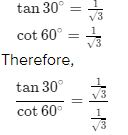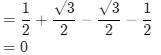Friday, March 24, 2023

# ML Aggarwal Trigonometrical Ratios of Standards Angles MCQs Class 9 ICSE Maths Solutions

ML Aggarwal Trigonometrical Ratios of Standards Angles MCQs Class 9 ICSE Maths APC Understanding Solutions. Solutions of  MCQs . This post is the Solutions of  ML Aggarwal Chapter 18 – Trigonometrical Ratios of Standards Angles for ICSE Maths Class-9.  APC Understanding ML Aggarwal Solutions (APC) Avichal Publication Solutions of Chapter-18 Trigonometrical Ratios of Standards Angles for ICSE Board Class-9Visit official website CISCE for detail information about ICSE Board Class-9.

## ML Aggarwal Trigonometrical Ratios MCQs Class 9 ICSE Maths Solutions

 Board ICSE Publications Avichal Publishig Company (APC) Subject Maths Class 9th Chapter-18 Trigonometrical Ratios of Standards Angles Writer ML Aggarwal Book Name Understanding Topics Solution of MCQs Edition 2021-2022

### MCQs Solutions of ML Aggarwal for ICSE Class-9 Ch-18, Trigonometrical Ratios of Standards Angles

Note:- Before viewing Solutions of Chapter -18 Trigonometrical Ratios of Standards Angles Class-9 of ML AggarwaSolutions .  Read the Chapter Carefully. Then solve all example given in Exercise-18.1, Exercise-18.2, MCQs, Chapter Test.

### Trigonometrical Ratios of Standards Angles MCQs

ML Aggarwal Class 9 ICSE Maths Solutions

Page 437

#### Question 1. the value of tan 30/cot60 is

(a) 1/√2

(b) 1/√3

(c) √3

(d) 1= 1

#### Question 2. the value of (sin 45 + cos 45 ) is

(a) 1/√2

(b) √2

(c) √3/2

(d) 1

sin 45° + cos 45° = 1/√2 + 1/√2

⇒ sin 45° + cos 45° = 2/√2 = √2

Thus, sin 45° + cos 45° = √2.

#### Question 3. the value of tan² 30 – 4 sin² 45 is

(a) 1

(b) 7/3

(c) -5/3

(d) -11/3

tan ²30° – 4 sin²45°

(1/√3)² – 4 × (1/√2)²

1/3 – 4 × 1/2

= -5/3

Trigonometrical Ratios of Standards Angles MCQs

### ML Aggarwal Class 9 ICSE Maths Solutions

Page 438

(a) 1/√2

(b) √3/2

(c) 1/2

(d) 1

2×sin30×cos30

2×1/2×√3/2

=√3/2

#### Question 5. The value of (sin 30 + cos 30) – (sin 60 + cos 60) is

(a) -1

(b) 0

(c) 1

(d) 2

sin 30 + cos 30) – (sin 60 + cos 60)(a) 0

(b) 1

(c) 2

(d) -1

√3 x 2/√3 – 2

= 0

#### Question 7. The value of 1/sin30 – √3/ cos 30 is

(a) 2

(b) 1

(c) 1/2

(d) 0

sin30°=1/2

cos30°= √3/2

1/sin30° = 1/1/2 = 2 …(eq1)

√3/cos30° = √3/√3/2 = 2 …(eq2)

subtracting eq2 from eq1, we get,

2–2=0

#### Question 8. If tan A = √3, then the value of cosec A is

(a) 1/2

(b) 2

(c) 1/√2

(d) √3/2

tan A=√3 ——-1

But, tan 60°=√3 ———-2

From 1 and 2, A=60°

cosec A=cosec 60°

=2/√3

#### Question 9. If sec θ.sin θ = 0, then the value of cos θ is

(a) 0

(b) 1/√2

(c) 1/2

(d) 1

Sec A × sin A = 0

( 1/cos A ) × sin A = 0

( Sin A/Cos A ) = 0

tan A = 0

tan A = tan 0°
Therefore ,
A = 0°
Now ,
Cos A = Cos 0° = 1

#### Question 10. If sin alpha = 1/2 then the value of 3 cos alpha – 4 cos³ alpha is

since sin alpha is = 1/2

sin 30 = 1/2

so,

alpha = 30

now,

3 cos30 – 4 cos³ 30

=> 3(√3)/2 – 4(√3/2)³

=> 3 x √3/2 – 4(3 x √3)/8

=> 12 x √3/8 – 12 x √3/8                       [taking common denominator]

=> 0

#### Question 11. The value of 1-tan² 45/ 1+ tan² 45 is equal to

(a) tan 60

(b) tan 30

(c) sin 45

(d) tan 0

1-tan² 45/ 1+ tan² 45

1-1/1+1

0/2

1-tan² 45/ 1+ tan² 45 = 0

tan 0

#### Question 12. if sin alpha = 1/2 and cos beta = 1/2, then the value of (alpha + beta) is

(a) 0

(b) 30

(c) 60

(d) 90

Sin alpha = 1/2
Sin alpha = sin 30°
Alpha = 30°
Cos alpha = 1/2
Cos beta = cos 60°
Beta = 60°
Now, alpha + beta
= 30°+60°
= 90°

#### Question 13. If triangle ABC is right angled at C, then the value of cos (A + B) is

(a) 0

(b) 1

(c) 1/2

(d) √3/2

since ABC is right angled and angle C is 90°

therefore,

A+B=180° – C

A+B=180°-90°

A+B= 90°

Hence, cos (A+B)=cos90°

=0

#### Question 14. In the adjoining figure, ABC is a right triangle right angled at B. If AB = 10 cm and angle C = 30°, then the length of the side BC is

(a) 10/√3 cm

(b) 10√3 cm

(c) 20 cm

(d) 5 cm

In the given
Sum of all angles is

#### Question 15. In the adjoining figure, PQR is a right triangle right angled at Q. If PQ = 4 cm and PR = 8 cm then angle P is equal to

(a) 60

(b) 45

(c) 30

(d) 15

cos P = PQ/PR

cos P = 4/8

= 1/2

cos P = 1/2

cos P = cos 60

so, P = 60

—  : End of ML Aggarwal Trigonometrical Ratios of Standards Angles MCQs Class 9 ICSE Maths Solutions :–

Thanks

RELATED ARTICLES